### Presenting Brian Breaking Badger

Hi, I’m Brian Breaking Badger, and I love to break numbers apart.
Separating place value is considered my art!
I break numbers into ones and tens with my teeth,
Then I work with the place value underneath.
I’ll add or subtract the tens, then the ones.
Before you know it, the problem’s all done!

WHAT is breaking apart? Students use place value knowledge to decompose or break each number apart into hundreds, tens and ones.  Depending on the problem, students will either add or subtract each place value (first hundreds, then tens, and finally ones).  Students will then add or subtract all numbers to solve the problem. Read more about Brian’s strategy below or download the complete unit here.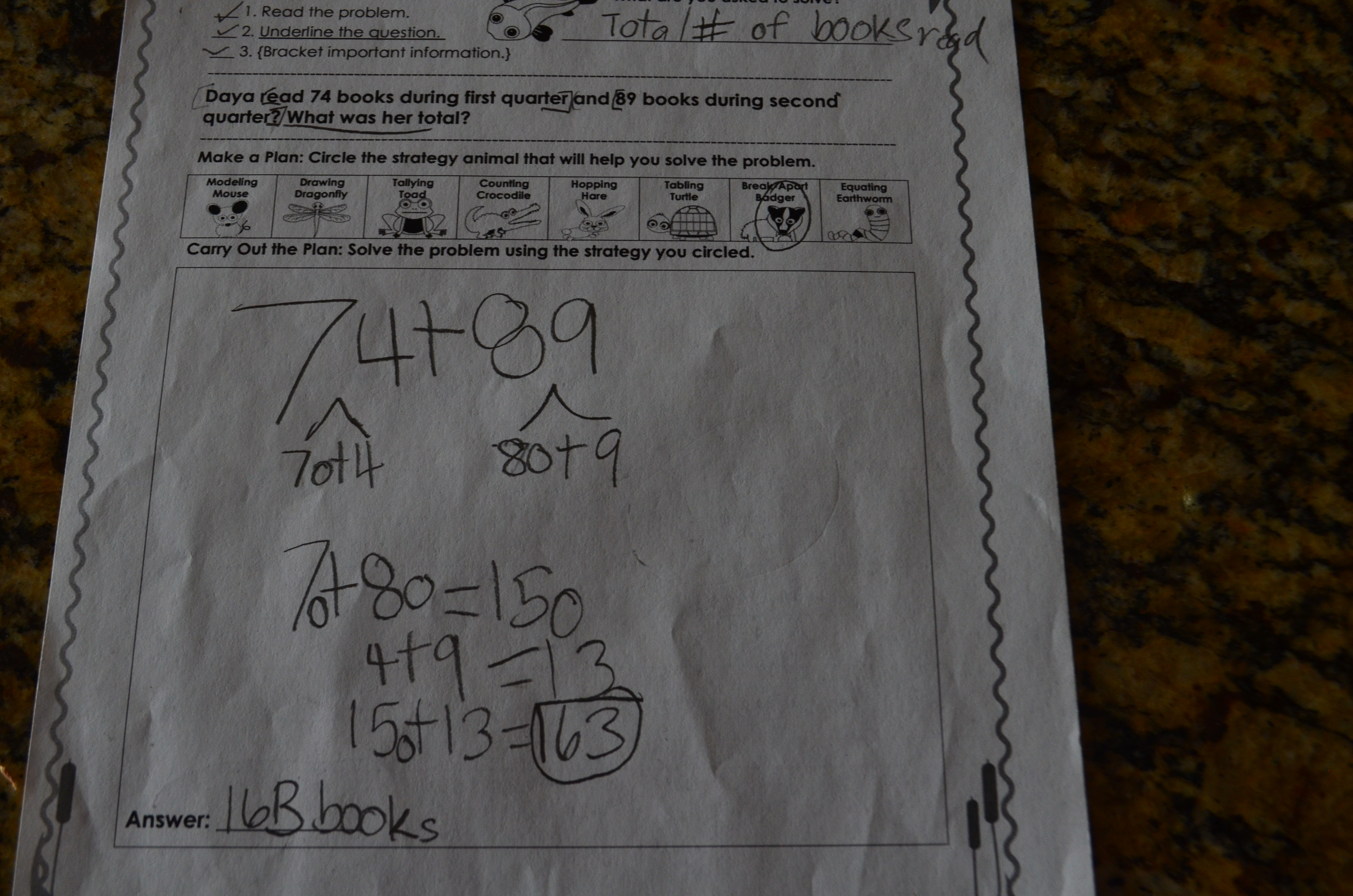WHY is breaking apart important?  When students use the breaking apart strategy, they are decomposing numbers by place value. This help helps strengthen mental computation, builds number sense and solidifies foundational place value skills. It also serves as an efficient method to double-check solutions as students.

HOW do I teach breaking apart? Teach breaking in isolation first so that students become familiar with the process of decomposing numbers. After proficiency is demonstrated, students can apply this strategy with story problems.

WHEN should I use breaking apart? This is an ideal strategy for developing mathematicians who have a solid place value and number sense foundation. It is most effective to introduce the breaking apart strategy after students are proficient with the hopping strategy since hopping requires place value identification and decomposition skills.

Provide place value and expanded notation practice. Students need a strong place value foundation to decompose or break apart numbers.  Provide regular practice through center games and kinesthetic activities. Simple games such as “Race to 100” where students roll dice and add numbers using place value manipulatives are engaging and effective.  This game is the perfect addition to weekly math centers.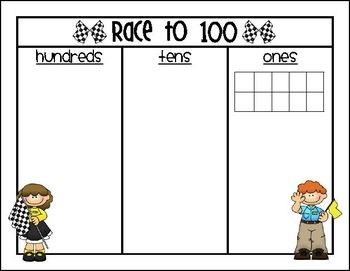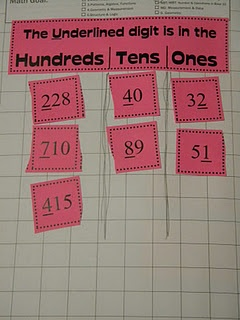Act out the problem.  Increase students’ understanding of the mathematical context by acting out the story problem.  Students also love to show their badger fangs when they break apart the numbers.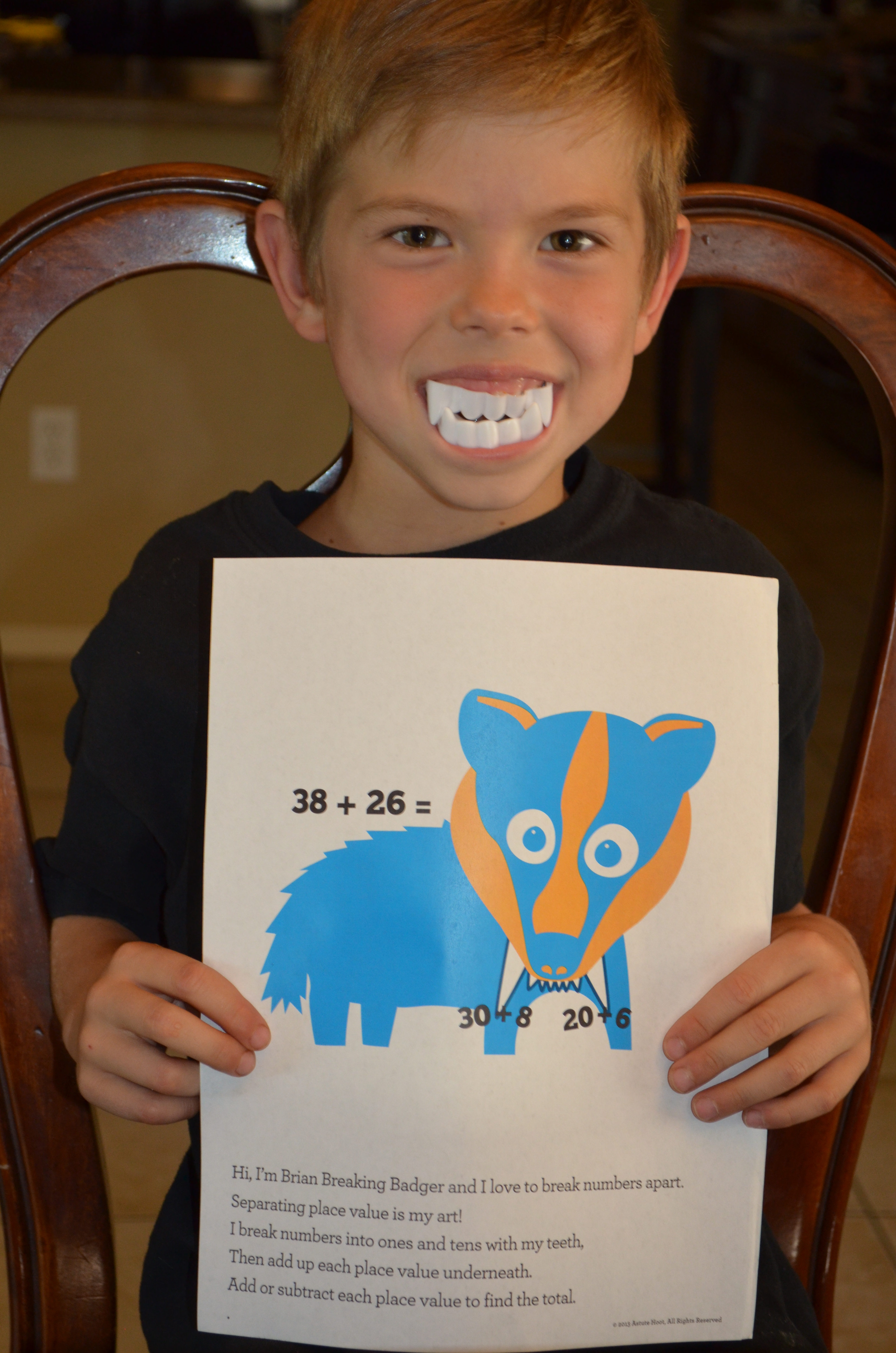### Introducing Daphne Drawing Dragonfly

Hi, I’m Daphne the Drawing Dragonfly.
Draw a mathematical picture to give my strategy a try.
Read the problem and draw what’s going on;
Then write a number sentence for what you have drawn.

Daphne Drawing Dragonfly is our newest animal in our Problem-Solving Pond: A Common Core Math Strategy Unit. The Problem-Solving Pond was created to help teachers overcome Common Core math challenges and employ problem-solving strategies with confidence and fidelity. Daphne’s unit is perfect for general education, special education, RTI and math intervention. Read more about Daphne’s strategy below or download the complete unit here.

WHAT is drawing? Students make a visual representation of the story problem such as a picture, bar model, tens frame or array.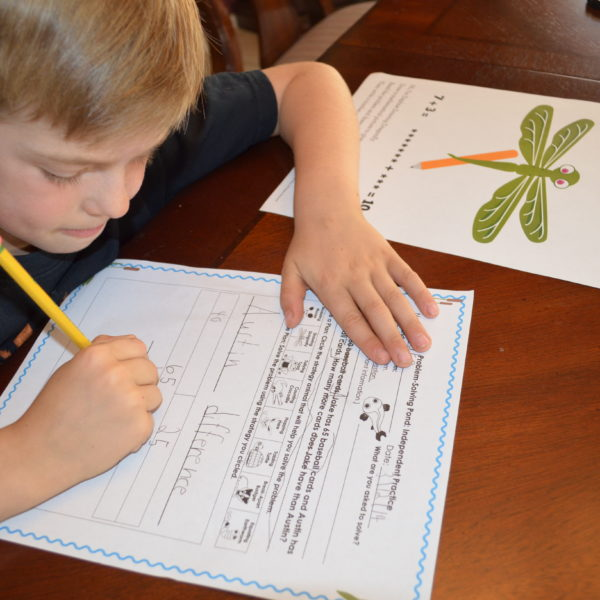WHY is drawing important? When students use the drawing strategy, they are making a concrete representation, strengthening understanding of the mathematical concepts.  Effective math classrooms include frequent use of pictorial representations to help students process and visualize mathematical concepts learned.

HOW do I teach drawing?Teach students to create neat, organized drawings with labels and numbers. Students will need to be taught the bar model and arrays, but it is best to let students create the pictorial representation that they see and works for them.

WHEN should I use drawing? This strategy is ideal for presenting a new mathematical operation.  Most teachers use this strategy with K-2 mathematicians, but this is also beneficial for older students as it works especially well with money, fractions, ratios and percentages. Drawing is a great way to double-check solutions because the visual representation increases understanding of the problem.

• Create drawing guidelines. As a class, decide important components to include in a mathematical drawing. Possible components include:
• Simple drawings
• Appropriate spacing
• Neat labels (words or numbers)
• Easy to read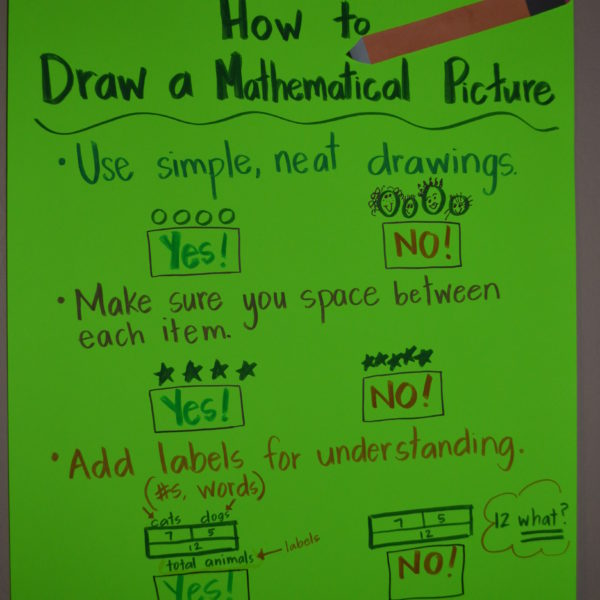• Encourage students to use a variety of pictorial representations.  Often students will get in a “strategy rut”, relying on one strategy for many different kinds of story problems. While drawing is an effective strategy for most problems, the type of pictorial representation used depends on the type of story problem.